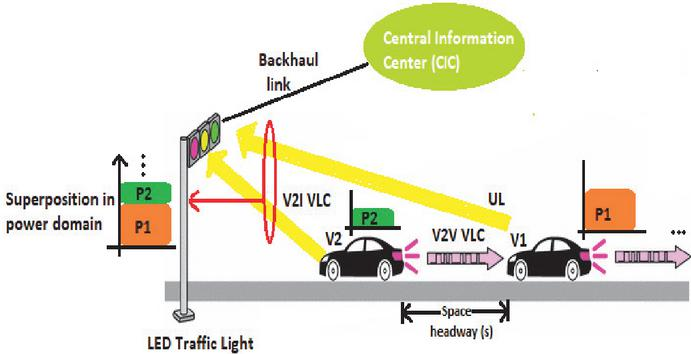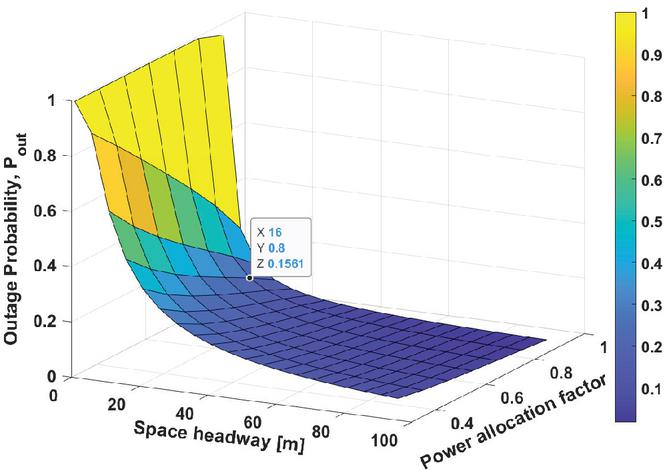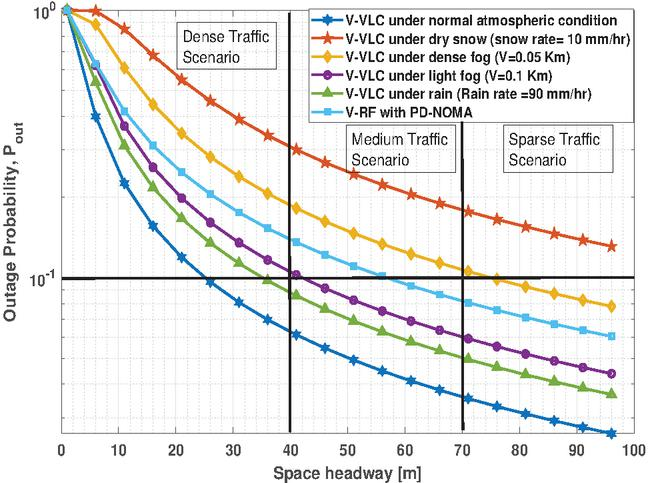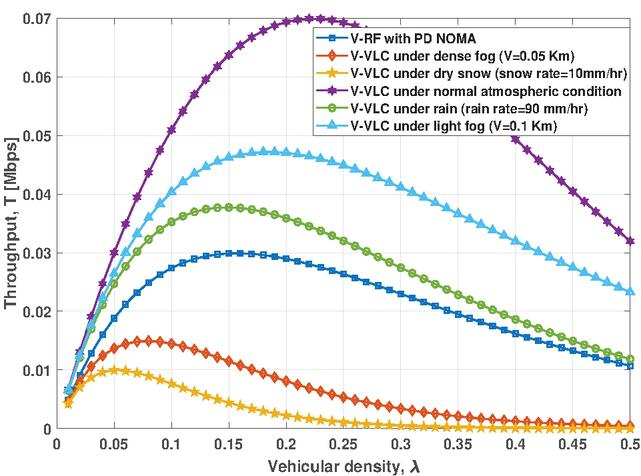Comparison of PD-NOMA for RF and VLC Based Vehicular Communication Under Various Weather Conditions

Gurinder Singh1,*, Anand Srivastava1, Vivek Ashok Bohara1 and Zilong Liu2

1Department of Electronics and Communications Engineering, IIIT-Delhi,

New Delhi-110020, India

2School of Computer Science and Electronic Engineering, University of Essex, UK

E-mail: gurinders@iiitd.ac.in; anand, vivek.b@iiitd.ac.in; zilong.liu@essex.ac.uk

*Corresponding Author

Received 10 July 2020; Accepted 01 September 2020; Publication 15 February 2021

## Abstract

In order to provide vehicles with reliable, ubiquitous, and massive connectivity, an appropriate multiple access (MA) scheme should be adopted. An appealing MA scheme referred to as non-orthogonal multiple access (NOMA) has been gaining significant attention in vehicular networks among academia and industry. This work aims to show the technical feasibility of uplink vehicular-VLC system (V-VLC) using OPD-NOMA, which is a promising technique for future intelligent transportation system which has not been explored before. We aim to present a comprehensive qualitative and quantitative analysis on the performance of Optical Power Domain-NOMA (OPD-NOMA) based Vehicular Visible Light Communication (V-VLC) systems. In particular, we aim for designing V-VLC systems with robust information exchange over various weather conditions such as rain, light fog, dense fog, dry snow, etc. We make use of various analytical tools of stochastic geometry to derive theoretical expression for outage probability and throughput by modelling the location of the vehicle as a spatial Poisson point process. We compare the performance of OPD-NOMA based V-VLC with Vehicular Radio Frequency (V-RF) communication for three different traffic scenarios (i.e., sparse, medium, and dense traffic) in terms of outage probability and throughput. Our numerical results reveal that instead of viewing V-VLC as competing technology, it can be visualized as complementary technology to V-RF technology under different environmental conditions for future Intelligent Transportation System (ITS) to meet diverse requirements of 5G networks and beyond.

## 1 Introduction

According to global status report on road safety, 2018 issued by World Health Organization (WHO), the number of annual road traffic deaths has reached 1.35 million. To address this global issue, various intelligent transportation system (ITS) applications could be adopted to prevent and reduce road accidents by improving Cooperative Awareness Messages (CAMs) or Basic Safety Messages (BSMs) dissemination. Currently, the exchange of CAMs/BSMs among moving vehicles (V2V) and roadside infrastructure (V2I) primarily utilize traditional Radio Frequency (RF) based V2X technology. However, in relation to meet the growing demands for future ITS, the currently used RF spectrum is insufficient. Further, conventional RF based vehicular networking tends to become incompetent in dense traffic scenarios as it may suffer from higher interference, longer communication delays, and lower Packet Reception Probability (PRP) when hundreds of vehicles located in the same vicinity try to communicate simultaneously.

Against this background, Visible Light Communication (VLC) offers an economically viable solution to implement Vehicle-to-everything (V2X) communications. Vehicular-Visible Light Communication (V-VLC) provides optical communication among vehicles using low-cost Light-Emitting Diodes (LEDs) and photo diodes. The use of optical bands complementary to RF may relieve the problems caused by spectrum crunch in RF-based wireless communication . Unlike RF based wireless communication, a critical research issue in the deployment of V-VLC is how to provide robust information exchange under adverse attenuating weather conditions such as light fog, dense fog, rain, dry snow, and wet snow. The impact of weather condition has received insufficient attention in literature so far.

In order to handle next generation vehicular network requirements supporting reliable massive connectivity and reduced resource collision, an appropriate multiple access (MA) scheme should be adopted. Recently, Non-Orthogonal Multiple Access (NOMA) scheme is emerging as a favourable multiple access scheme for next generation cellular networks. Compared to other multiple access techniques, NOMA provides higher spectral efficiency, better connectivity, user fairness, reduced latency and enhanced data rates which makes it a viable candidate for future development of vehicular communication systems. NOMA allows multiple users to share the same channel resource via power domain or code domain multiplexing. Authors in  introduced NOMA techniques in power and code domains for LTE-based vehicular networks to support reduced resource collision and massive connectivity. Power domain NOMA allocates considerably higher power to the vehicle with worse channel conditions, which improve system throughput and fairness.

Apart from its applications in conventional RF based communications, the performance of Optical Power Domain-NOMA (OPD-NOMA) based VLC systems has been widely investigated in [5, 6, 7, 8, 9]. PD-NOMA  multiplexes the users in power domain and applies the iteration-based Successive Interference Cancellation (SIC) to detect multiple signal streams at the receiver [11, 12]. Authors in  have shown that PD-NOMA is capable of improving the resource utilization efficiency in both uplink and downlink channels.

To quantify the impact of weather conditions on the performance of OPD NOMA based V-VLC and V-RF communication, we make use of various analytical tools of stochastic geometry. Stochastic geometry [13, 14] can be used to characterize the randomness in the positions of vehicles. It is indeed a powerful tool for random interference modeling and performance analysis, with applications in numerous types of vehicular adhoc networks (VANET) . The work in [16, 17] applies stochastic geometry in VANET to determine the average successful transmission rate assuming that road vehicles are spatially distributed according to a linear Poisson point process (PPP).

To the best of authors’ knowledge, there has been no previous works on PD-NOMA based V-VLC systems to evaluate the reliability of packet transmission using stochastic geometry. We aim to present a comprehensive qualitative and quantitative analysis on the performance of PD-NOMA based V-VLC in presence of adverse weather conditions. We make use of various analytical tools of stochastic geometry. We compare the performance of PD-NOMA based V-VLC for three different traffic scenarios (i.e., sparse, medium, dense traffic) in terms of outage probability and throughput as a performance metric under different weather conditions, e.g., light fog, dense fog, dry snow and rain. Our numerical results show that V-VLC with OPD-NOMA technique under normal atmospheric condition provides a more reliable option for dense traffic scenarios. While, V-RF communication is rather more suitable option for vehicular communication as compared to V-VLC under dense fog or dry snow conditions.

The organization of the paper is as follows: Section 2 presents the system model and various assumptions made for our analysis. We derive the theoretical expression of outage probability and throughput using tools of stochastic geometry in Section 3. In Section 4, the numerical results and discussion are provided with useful insights. Finally, concluding remarks and future scope of current work are given in Section 5.Figure 1 System model consisting of uni-directional traffic stream. The transmitting vehicle of interest can be at any location, while LED traffic light acts as target receiver. It is assumed that only proportion of vehicles, $ϱ$ transmits concurrently and causes interference.

## 2 System Model

### 2.1 Network Model

For sake of analysis, we consider uni-directional traffic stream wherein either VLC or RF uplink exists between vehicles and Road Side Unit (RSU) (mounted on LED traffic lamp) as depicted in Figure 1. The space headway ($s$) denotes inter-vehicular distance between vehicles. In traffic flow theory, it is also defined as reciprocal of vehicular density, $λ$. The vehicular density, $λ$ denotes the number of vehicles per lane per km. We consider the existence of a central information center (CIC) that collects and keeps track of the necessary information (such as location and speed of vehicle, road condition, BSMs dissemination etc) about the on road vehicles. The communication between LED Traffic light and CIC is established via back-haul connectivity and to vehicles through free-space wireless transmission over the light signals. At a given time instant, we assume there is a fraction $ϱ$ of vehicles that are active, which means they are transmitting, and a fraction $1-ϱ$ of vehicles that are non-active, which means they are potentially receiving signals from LED Traffic light or does not have any information to transmit. We further assume that the probability of any vehicle being active is independent of all the other vehicles. This approach results in a Poisson process of active transmitting vehicles with density $ϱ⁢λ$. We incorporate complete randomness in the location of vehicles in each lane by modelling it as a one-dimensional homogeneous PPP, $ΨP⁢P⁢P$ with vehicular density, $λ$. The received signal at RSU from $N$ transmitting vehicles can be given as

 $y=∑k=1Nhk⁢sk+n,$ (1)

where $hk$ denotes the channel gain associated with vehicle-$k$, $n$ denotes the additive white Gaussian noise (AWGN) with a zero mean and variance $σk2$. We assume that there is a perfect interference cancellation through SIC at the receiver.

### 2.2 Channel Model for VLC and RF

For a VLC system, the direct current (DC) gain of a VLC channel is expressed by 

 $hk=(m+1)⁢AR2⁢π⁢Dkγ⁢cosm⁡(ϕk)⁢cos⁡(Ψk)⁢Ts⁢(Ψk)⁢g⁢(Ψk),$ (2)

where, $AR$, $ϕk$ and $Ψk$ denote the area of PD, the angle of irradiance and the angle of arrival (AoA) respectively. $Ts⁢(Ψk)$ denotes gain of the optical filter at the receiver; $g⁢(Ψk)$ denotes the gain of optical concentrator at the receiver front-end which is given as

 $g⁢(Ψk)={n2sin2⁡ΨF⁢O⁢V;if⁢ 0≤ϕk≤ΨFOV,0;if⁢ϕk>ΨFOV,$ (3)

where n denotes the refractive index of the optical concentrator; and $Dk$ denotes the Euclidean distance between the $kt⁢h$ vehicle and RSU. Here, m is the order of the Lambertian model and is given by $m=-ln⁡(2)ln⁡(cos⁡(ϕ12))$. Given a RF link, fading gain ($hk$) is an exponential random variable (r.v.) of unit mean in the case of Rayleigh fading.

### 2.3 Power Allocation Strategy and Practical Challenges

For transmitting information in power domain, recognising that $Vi=1,2,…⁢n$ has worse channel conditions, PD-NOMA allocates a greater amount of power $Pi=1,2,…⁢n$ to that vehicle. A lower power (for instance, $P2) is allocated to $Vi$ (here, $V2$) with higher channel gain. The overall transmit power $Pt$ is divided among vehicles based on power allocation strategy and channel state condition as follows

 $P1=ξ1⁢Pt,P2=ξ2⁢Pt,∑i=1nξi=1.$ (4)

where $Pt$ denotes transmission power and $ξi$ represents the power allocation coefficient associated with vehicle-$i$.

Such non-uniform transmit power allocation strategy adopted among vehicles is critical for designing a practical V-VLC or V-RF system. Several open issues such as power imbalance and maintaining fairness among vehicles need to be addressed carefully before practical deployment of PD-NOMA to vehicular communication systems. In order to ensure practical feasibility of proposed scenario, we assume that each vehicle is allocated power more than certain minimum threshold power which can suffice illumination as well as communication constraint.

## 3 Performance Analysis

### 3.1 Outage Probability

We characterize the performance of the VLC based vehicular communication in the presence of the aggregate interference and AWGN in terms of outage probability as a performance metric. An outage occurs when the SINR falls below a given SINR threshold, $β$. Mathematically,

 $Po⁢u⁢t,V⁢L⁢C⁢(β)=ℙ(𝒮ℐ𝒩ℛ<β),=ℙ(𝒮ℐ+σ2<β).$ (5)

Based on simple geometrical argument, one can find $cos⁡(ϕ)=cos⁡(ψ)=xh2+x2$ for given system model. Here, $h$ denotes the height of RSU. The desired signal power $𝒮$ and interference $ℐ$ can be defined as

 $𝒮=k⁢D2⁢(m+1)(h2+D2)(m+γ+1)⁢ξ⁢PV⁢L⁢Cℐ=∑i=1Nk⁢xi2⁢(m+1)(h2+xi2)(m+γ+1)⁢(1-ξ)⁢PV⁢L⁢C$ (6)

Here $k=((m+1)⁢AR2⁢π⁢Ts⁢(ψ)⁢G⁢(ψ))2$. In above, $PV⁢L⁢C$ and $D$ denote the transmission power for V-VLC and distance between legitimate vehicle and RSU respectively. The electrical SINR can be represented as:

 $𝒮⁢ℐ⁢𝒩⁢ℛ=1ℐk⁢Zo⁢ξ⁢PV⁢L⁢C+1α0$ (7)

where, $α0=k⁢Zo⁢ξ⁢PV⁢L⁢Cσ2$ and $Zo=D2⁢(m+1)(h2+D2)(m+γ+1)$. By definition of outage probability,

 $Po⁢u⁢t=ℙ(ℐk⁢Zo⁢ξ⁢PV⁢L⁢C+1α0>1β)$ (8)

We define random variable W as:

 $W=ℐk⁢Zo⁢ξ⁢PV⁢L⁢C+1α0$ (9)

Hence, Equation (8) can be rewritten as

 $Po⁢u⁢t,V⁢L⁢C⁢(β)=ℙ(W>β-1)=1-FW(β-1).$ (10)

In general, it is quite difficult to obtain a closed-form solution for $FW⁢(β-1)$. Hence, we make use of numerical inversion of Laplace transform to find CDF, $FW⁢(β-1)$. The CDF of a random variable $W$ is related to the Laplace transform of $FW⁢(w)$ as

 $FW⁢(w)=12⁢π⁢j⁢∫c-j⁢∞c+j⁢∞ℒFW⁢(w)⁢exp⁡(s⁢w)⁢ds.$ (11)

where $j$ denotes imaginary unit ($-1$). The above integral can be discretized to get a series using the trapezoid rule and then the infinite series can be truncated to get a finite sum using the Euler summation . Also, $ℒFW⁢(w)⁢(s)=ℒW⁢(s)s$. Moreover, (10) can be approximated as

 $Po⁢u⁢t,V⁢L⁢C⁢(β)$ $≈1-2-B⁢exp⁡(A2)β-1$ $×∑b=0B(Bb)⁢∑c=0C+b(-1)cDc⁢R⁢e⁢{ℒW⁢(s)s}.$ (12)

where $Dc=2$ (if $c$ = 0) and $Dc=1$ (if $c=1,2,3,…$) and $s=(A+j⁢2⁢π⁢c)2⁢β-1$. The estimation error is controlled by three parameters $A$, $B$ and $C$. Using the well established result given in [21, 20], in order to achieve an estimation accuracy of 10$-η$ (i.e., having the $(η-1)$th decimal correct), $A$, $B$ and $C$ have to be at least equal to $η$ln10, 1.243$η-1$, and 1.467$η$, respectively. Setting $A=8$ln10, $B=11,C=14$ achieves stable numerical inversion with an estimation error of $10-8$.

Using the definition of the Laplace transform of the probability distribution of a random variable,

 $ℒW⁢(s)=𝔼ℐ⁢{exp⁡(-s⁢(ℐk⁢Zo⁢ξ⁢PV⁢L⁢C+1α0))}=𝔼x⁢{exp⁡(-sα0)⁢exp⁡(-s⁢ℐk⁢Zo⁢ξ⁢PV⁢L⁢C)}=exp⁡(-sα0)⁢𝔼x⁢{∏i=1Nexp⁡(-s⁢(1-ξ)⁢Zi⁢xi2⁢(m+1)Zo⁢ξ⁢(h2+xi2)(m+γ+1))}$ (13)

The expectation in Equation (13) can be solved using probability generating functional Laplace (PGFL) defined for a homogeneous Poisson point process [19, Th 4.9].

 $𝔼x⁢{∏i=1Nexp⁡(-s⁢(1-ξ)⁢Zi⁢xi2⁢(m+1)Zo⁢ξ⁢(h2+xi2)(m+γ+1))}=exp⁡[-ϱ⁢λ⁢∫0∞(1-exp⁡(-s⁢(1-ξ)⁢Z⁢xi2⁢(m+1)Zo⁢ξ⁢(h2+x2)(m+γ+1)))⁢dx].$ (14)

Regarding RF based vehicular communication, assuming free space path loss propagation model the interference at the receiver can be given as sum of all the power received from $N$ interferers as:

 $IR⁢F=∑x∈ΦP⁢P⁢PPR⁢F⁢Gt⁢Gr⁢ℓ⁢hx⁢||h2+x2||-α2,$ (15)

where $ℓ:=c2(4⁢π)2⁢f02$. In above expression, $PR⁢F$, $α$, $Gt$ and $Gr$ are the transmission power for V-RF, the path loss exponent, the antenna gains for transmitter and receiver respectively . The outage probability ($Po⁢u⁢t$) in case of RF based vehicular communication can be given as

 $Po⁢u⁢t,R⁢F⁢(ζ)=1-Ps⁢(ζ)$ (16)

The probability of successful transmission, $Ps⁢(ζ)$ can be calculated as

 $Ps⁢(ζ)=ℙ(𝒮ℐ𝒩ℛ>ζ),=ℙ(ξ⁢PR⁢F⁢Gt⁢Gr⁢ℓ⁢hx⁢D-αIR⁢F+σ2>ζ),=𝔼IR⁢F[ℙ(hx>ζξ⁢PR⁢F⁢Gt⁢Gr⁢ℓ⁢D-α(IR⁢F+σ2))],=exp⁡(-ζ⁢σ2ξ⁢PR⁢F⁢Gt⁢Gr⁢ℓ⁢D-α)×𝔼IR⁢F⁢[exp⁡(-ζ⁢(IR⁢F)ξ⁢PR⁢F⁢Gt⁢Gr⁢ℓ⁢D-α)],=exp⁡(-ζ⁢σ2ξ⁢PR⁢F⁢Gt⁢Gr⁢ℓ⁢D-α)⁢ℒIR⁢F⁢(ζξ⁢PR⁢F⁢Gt⁢Gr⁢ℓ⁢D-α)$ (17)

where, $ℒ(.)$ stands for Laplace transform which is given as1 

 $ℒIR⁢F⁢(ζξ⁢PR⁢F⁢Gt⁢Gr⁢ℓ⁢D-α)=exp⁡(-ϱ⁢λ⁢((1-ξ)⁢ζξ)1α⁢D⁢πα⁢csc⁡(πα)).$ (18)

### 3.2 Spatial Throughput

The spatial throughput is an outage-based metric. The probability of successful transmission can be viewed as a throughput metric for a particular link. The throughput is defined as the product of the probabilistic spatial throughput and the rate of transmission, assuming that capacity-achieving codes are used. Mathematically,

 $𝒯⁢(ϱ)=ϱ⁢λ⁢(1-Po⁢u⁢t)⁢l⁢o⁢g2⁢(1+β)⁢Bs.$

## 4 Numerical Results and Discussion

In this section, we present numerical results that substantiate our theoretical findings. The system parameters for V-VLC and V-RF communication have been summarized in Table 1.

Table 1 System model parameters

 Parameter Symbol Value Lambertian Order $m$ 2 PD active detection area $AR$ 1 $c⁢m2$ PD’s field of view (FOV) $ΨFOV$ 53$∘$, 28$∘$, 14$∘$ LED semi-angle $Φ12$ 70$∘$ Transmission power for V-VLC $PV⁢L⁢C$ 36.5 dBm Transmission power for V-RF $PR⁢F$ 23 dBm Responsivity of the PD $ℛ$ 0.54 A/W  Absolute temperature $Tk$ 298$∘$ K System Bandwidth $Bs$ 20 MHz Optical filter gain $Ts⁢(Ψk)$ 1 Refractive index n 1.5 Power allocation coefficient $ζ$ 0.5-0.9 Height of RSU $h$ 10 m Attenuation coefficient under dense fog (V $=$ 0.05 km) $β$ 78.8 dB/Km [24, 25] Attenuation coefficient under light fog (V $=$ 0.1 km) $β$ 39.4 dB/Km [24, 25] Attenuation coefficient under rain (rain rate $=$ 90 mm/hr) $βr⁢a⁢i⁢n$ 21.9 dB/Km  Attenuation under dry snow (snow rate $=$ 10 mm/hr) $βs⁢n⁢o⁢w$ 131 dB/Km 

The cumulative effect of traffic intensity, $λ$ and power allocation factor, $ξ$ for PD-NOMA V-VLC can be observed from Figure 2. The outage performance improves significantly with increasing space headway between vehicles and power allocation factor. The outage probability, $Po⁢u⁢t$ is higher for high traffic density scenario. For such a high traffic density scenario, PD NOMA ($ξ=0.8$) based V-VLC under normal atmospheric condition provides packet reception probability of around 84$%$ and seems a more reliable option for communication among vehicles as compared to V-RF communication. The decrease in average received optical power under various weather conditions becomes more severe such that V-RF becomes a more reliable option for communication among vehicles as compared to V-VLC, especially under dense fog or dry snow condition. For ease of visualization and validation, the analytical results for PD-NOMA based V-RF and V-VLC under various weather conditions has been shown in Figure 3.Figure 2 3D surface plot for outage probability as a function of space headway and power allocation factor, : V-VLC under normal atmospheric condition.Figure 3 Outage performance of PD-NOMA based V-RF and V-VLC under various weather attenuating conditions for three different traffic scenario.Figure 4 Throughput variation against space headway for PD NOMA based V-RF and V-VLC under various weather attenuating conditions.

Figure 3 shows analytical outage results for PD-NOMA based V-RF and V-VLC under various weather conditions for three different traffic scenario. Here, power allocation factor, $ξ$ and communication distance between legitimate vehicle and RSU, D are kept to be 0.8 and 100 m respectively. With outage probability of below 10$%$ as a performance benchmark, V-VLC under normal atmospheric condition, rain and light fog condition has a potential benefit over conventional V-RF communication supporting dense traffic scenario. However, it is worth mentioning here that V-RF can be a more reliable option for vehicular communication supporting medium traffic scenario as compared to V-VLC under dense fog or dry snow condition, ensuring outage probability of less than 10$%$ for a particular link.

In Figure 4, we plot the throughput with respect to vehicular density, $λ$ for PD-NOMA based V-RF and V-VLC under various weather attenuating conditions. Here, power allocation factor, $ξ$ and communication distance between legitimate vehicle and RSU, $D$ are kept to be 0.8 and 100 m respectively. It should be noted here that the throughput first rises (due to more active transmitting vehicles) and then decreases (overwhelming amount of interference) with increase in vehicular density, $λ$. Therefore, there also exists an optimal vehicular density that maximizes the overall system throughput. In addition, the maximum throughput can be achieved for V-VLC under normal atmospheric condition. While, V-RF communication achieves higher throughput as compared to V-VLC under dense fog or dry snow conditions.

## 5 Concluding Remarks

The proposed work shows the potential benefit of employing VLC for vehicular communication over conventional V-RF under unfavourable weather conditions. The presented framework also show the limitation of V-VLC under dense fog or dry snow conditions over V-RF communication. Our numerical results show that V-VLC may be visualized as a complementary technology to V-RF technology under adverse weather conditions to meet diverse requirements for future ITS.

It may be noted that the proposed work is just a preliminary step to understand the performance of PD-NOMA based V-RF and V-VLC under adverse weather conditions. Several open research challenges such as power imbalance among vehicles, impact of channel symmetry, power allocation techniques under feedback delay, synchronization in a high-mobility scenario, non linear distortion in OPD-NOMA, co-existence of V-VLC and V-RF, etc still needs to be explored. We hope that this comparative analysis may serve as a valuable resource for future invention and development of VLC based vehicular networks.

## Acknowledgment

This publication is an outcome of the research and development (RD) work undertaken project under the Visvesvaraya PhD Scheme of Ministry of Electronics Information Technology, Government of India, being implemented by Digital India Corporation.

## Note

1The closed form expression was obtained based on assumption that height of traffic lamp can be ignored as compared to distance between legitimate vehicle and traffic lamp i.e h$<$$<$x

## References

 M. Z. Chowdhury, M. T. Hossan, A. Islam, and Y. M. Jang, “A Comparative Survey of Optical Wireless Technologies: Architectures and Applications,” IEEE Access, vol. 6, pp. 9819–9840, 2018.

 M. Elamassie, M. Karbalayghareh, F. Miramirkhani, R. C. Kizilirmak, and M. Uysal, “Effect of Fog and Rain on the Performance of Vehicular Visible Light Communications,” in 2018 IEEE 87th Vehicular Technology Conference (VTC Spring), June 2018, pp. 1–6.

 M. Liaqat, K. A. Noordin, T. A. Latef, and K. Dimyati, “Power-Domain Non Orthogonal Multiple Access (PD-NOMA) in Cooperative Networks: an Overview,” Wireless Networks, pp. 1–23, 2018.

 B. Di, L. Song, Y. Li, and Z. Han, “V2X meets NOMA: Non-Orthogonal Multiple Access for 5G-Enabled Vehicular Networks,” IEEE Wireless Commun., vol. 24, no. 6, pp. 14–21, Dec 2017.

 H. Marshoud, V. M. Kapinas, G. K. Karagiannidis, and S. Muhaidat, “Non-Orthogonal Multiple Access for Visible Light Communications,” IEEE Photonics Technology Letters, vol. 28, no. 1, pp. 51–54, Jan 2016.

 L. Yin, W. O. Popoola, X. Wu, and H. Haas, “Performance Evaluation of Non-Orthogonal Multiple Access in Visible Light Communication,” IEEE Transactions on Communications, vol. 64, no. 12, pp. 5162–5175, 2016.

 X. Guan, Q. Yang, Y. Hong, and C. C.-K. Chan, “Non-Orthogonal Multiple Access with Phase Pre-Distortion in Visible Light Communication,” Optics express, vol. 24, no. 22, pp. 25 816–25 823, 2016.

 Y. Fu, Y. Hong, L.-K. Chen, and C. W. Sung, “Enhanced Power Allocation for Sum Rate Maximization in OFDM-NOMA VLC systems,” IEEE Photonics Technology Letters, vol. 30, no. 13, pp. 1218–1221, 2018.

 C. Chen, W.-D. Zhong, H. Yang, and P. Du, “On the Performance of MIMO-NOMA-Based Visible Light Communication Systems,” IEEE Photonics Technology Letters, vol. 30, no. 4, pp. 307–310, 2017.

 N. DOCOMO, “Initial Views and Evaluation Results on Non-Orthogonal Multiple Access for NR,” in R1-165175, 3GPP TSG RAN WG1 Meeting, vol. 85, 2016.

 W. Roh, “5G mobile communications for 2020 and beyond-vision and key enabling technologies,” Key note: at IEEE WCNC, 2014.

 Y. Saito, Y. Kishiyama, A. Benjebbour, T. Nakamura, A. Li, and K. Higuchi, “Non-Orthogonal Multiple Access (NOMA) for Cellular Future Radio Access,” in 2013 IEEE 77th vehicular technology conference (VTC Spring).   IEEE, 2013, pp. 1–5.

 A. Al-Hourani, S. Kandeepan, and A. Jamalipour, “Stochastic Geometry Study on Device-to-Device Communication as a Disaster Relief Solution,” IEEE Transactions on Vehicular Technology, vol. 65, no. 5, pp. 3005–3017, 2015.

 A. Al-Hourani, S. Kandeepan, and E. Hossain, “Relay-Assisted Device-to-Device Communication: A stochastic analysis of Energy Saving,” IEEE Transactions on Mobile Computing, vol. 15, no. 12, pp. 3129–3141, 2016.

 Y. Wang, F. Liu, C. Wang, P. Wang, and Y. Ji, “Stochastic Geometric Analysis in Cooperative Vehicular Networks Under Weibull Fading,” IEEE Access, vol. 7, pp. 158 655–158 670, 2019.

 B. Błaszczyszyn, P. Mühlethaler, and Y. Toor, “Stochastic Analysis of Aloha in Vehicular Ad hoc Networks,” Annals of telecommunications-Annales des télécommunications, vol. 68, no. 1-2, pp. 95–106, 2013.

 B. Blaszczyszyn, P. Mühlethaler, and Y. Toor, “Maximizing Throughput of Linear Vehicular Ad-hoc Networks (VANETs)—A Stochastic Approach,” in 2009 European Wireless Conference. IEEE, 2009, pp. 32–36.

 T. Komine and M. Nakagawa, “Fundamental Analysis for Visible-Light Communication System using LED lights,” IEEE transactions on Consumer Electronics, vol. 50, no. 1, pp. 100–107, 2004.

 M. Haenggi, Stochastic Geometry for Wireless Networks.   Cambridge University Press, 2012.

 C. A. O’cinneide, “Euler Summation for Fourier series and Laplace Transform Inversion,” Stochastic Models, vol. 13, no. 2, pp. 315–337, 1997.

 J. Abate and W. Whitt, “Numerical Inversion of Laplace Transforms of Probability Distributions,” ORSA Journal on computing, vol. 7, no. 1, pp. 36–43, 1995.

 A. Goldsmith, Wireless Communications. Cambridge university press, 2005.

 E. Steinmetz, M. Wildemeersch, T. Q. S. Quek, and H. Wymeersch, “A stochastic geometry model for vehicular communication near intersections,” in 2015 IEEE Globecom Workshops (GC Wkshps), 2015, pp. 1–6.

 G. Singh, A. Srivastava, and V. A. Bohara, “Impact of Weather Conditions and Interference on the Performance of VLC based V2V Communication,” in 2019 21st International Conference on Transparent Optical Networks (ICTON), July 2019, pp. 1–4.

 G. Singh, A. Srivastava, and V. A. Bohara, “On Feasibility of VLC Based Car-to-Car Communication under Solar Irradiance and Fog Conditions,” in Proceedings of the 1st International Workshop on Communication and Computing in Connected Vehicles and Platooning, ACM MOBICOM, 2018, pp. 1–7.